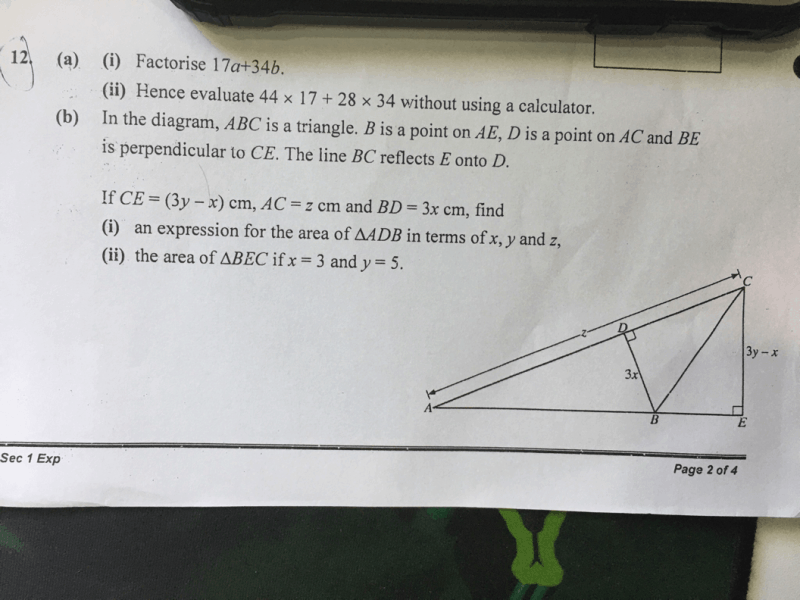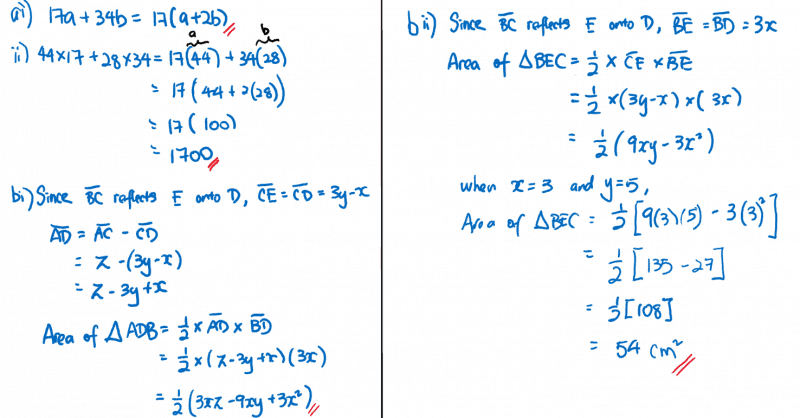# QuestionHelp pls.

thanks.

Q12

a)

i)

17a+34b

=17(a+2b)

ii)

44×17+28×34
=17[44+2(28)]
=17(44+56)
=17(100)

=1700

im not really sure how to do b(i) but I know b(ii)

If x=3 and y=5,

Length of CE

=3(5)-3

=12cm

BD=BE, so

length of BE

=3(3)

=9cm

area of triangle BEC

=1/2x12x9

=54cm2

0 Replies 0 Likes0 Replies 0 Likes